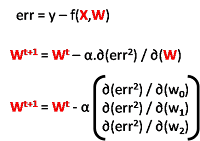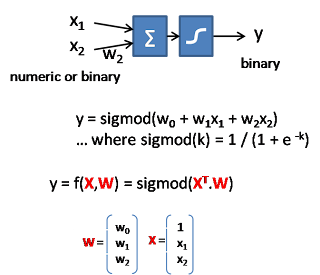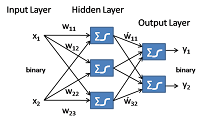## Friday, May 8, 2009

### Machine Learning: Linear Model

Linear Model is a family of model-based learning approaches that assume the output y can be expressed as a linear algebraic relation with the input attributes x1, x2 ...

Here our goal is to learn the parameters of the underlying model, which the coefficients.

Linear RegressionHere the input and output are both real numbers, related through a simple linear relationship. The learning goal is to figure out the hidden weight value (ie: the W vector).

Given a batch of training data, we want to figure out the weight vector W such that the total sum of error (which is the difference between the predicted output and the actual output) to be minimized.Instead of using the batch processing approach, a more effective approach is to learn incrementally (update the weight vector for each input data) using a gradient descent approach.

Gradient DescentGradient descent is a very general technique that we can use to incrementally adjust the parameters of the linear model. The basic idea of "gradient descent" is to adjust each dimension (w0, w1, w2) of the W vector according to their contribution of the square error. Their contribution is measured by the gradient along the dimension which is the differentiation of the square error with respect to w0, w1, w2.

In the case of Linear Regression ...Logistic RegressionLogistic Regression is used when the output y is binary and not a real number. The first part is the same as linear regression while a second step sigmod function is applied to clamp the output value between 0 and 1.

We use the exact same gradient descent approach to determine the weight vector W.Neural NetworkInspired by how our brain works, Neural network organize many logistic regression units into layers of perceptrons (each unit has both input and outputs in binary form).

Learning in Neural network is to discover all the hidden values of w. In general, we use the same technique above to adjust the weight using gradient descent layer by layer. We start from the output layer and move towards the input layer (this technique is called backpropagation). Except the output layer, we don't exactly know the error at the hidden layer, we need to have a way to estimate the error at the hidden layers.

But notice there is a symmetry between the weight and the input, we can use the same technique how we adjust the weight to estimate the error of the hidden layer.Support Vector Machine